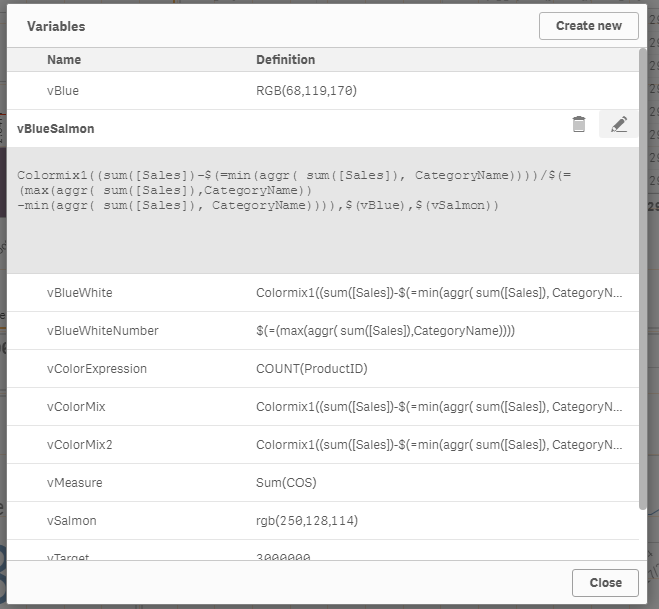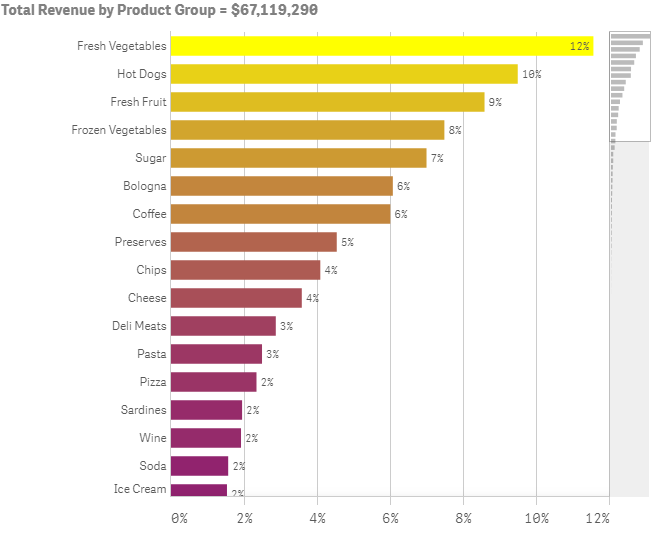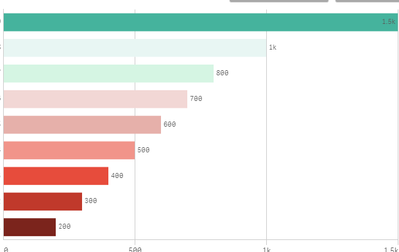# New to Qlik Sense

Discussion board where members can get started with Qlik Sense.

Announcements
Talk to Experts, a LIVE Q&A Webinar. Bring your Qlik Sense Business questions on Aug. 4th. Register
cancel
Showing results for
Did you mean:
HighlightedSpecialist

## Re: Change Color by Measure - Qlik SenseHighlightedContributor II

## Re: Change Color by Measure - Qlik Sense

I always seem to find this thread when trying to figure out how to adjust the colors for a gradient by measure. So I'm posting this example code here. I tried to make it  pretty simple so I can just change it up when I have a new chart:

Colormix1((

[Expression]

-\$(=min(aggr(

[Expression]

,

[Category]

))))

/\$(=(max(aggr(

[Expression]

,

[Category]

))

-min(aggr(

[Expression]

,

[Category]

)))),

[min_color]

,

[max_color]

)

How I used it:

Colormix1((

sum([Sales Quantity]*[Sales Price])

-\$(=min(aggr(

sum([Sales Quantity]*[Sales Price])

,

[Product Sub Group Desc]

))))

/\$(=(max(aggr(

sum([Sales Quantity]*[Sales Price])

,

[Product Sub Group Desc]

))

-min(aggr(

sum([Sales Quantity]*[Sales Price])

,

[Product Sub Group Desc]

)))),

magenta()

,

yellow()

)HighlightedCreator III

## Re: Change Color by Measure - Qlik Sense

Please use the Colormix1 or colormix2 function to achieve this functionality.

More details and sample file available in the below link.

Qlik Sense Color Range Theme Approach

The approach aims to use an expression that doesn't need to be rewritten each time the measure changes and can be copied across different objects without changes.

HighlightedMaster

## Re: Change Color by Measure - Qlik Sense

Try like this, may be:

Here's what I did.  I made a bar chart...

First dimension:

Field: Segment

Label: Segment

Field: ValueList('Sales','Quota','Expenses')

Label: Account Type

Field:

if(ValueList('Sales','Quota','Expenses')='Sales'

,Sum(Sales)

,if(ValueList('Sales','Quota','Expenses')='Quota'

,Sum(Quota)

,Sum(Expenses)

)

)

Label: Amount

Sorting in this example is by Segment then by Account Type then by Amount, with Account Type as descending alphabetical.

Now to set the colors.  I went to "Appearance" then "Colors and Legend", then turned off Auto for Colors.  In the dropdown, selected "By expression".  I entered this expression:

if(ValueList('Sales','Quota','Expenses')='Sales'

,rgb(0,192,0)

,if(ValueList('Sales','Quota','Expenses')='Quota'

,rgb(0,0,192)

,rgb(192,0,0)

)

)

I made sure the check box was checked for "The expression is a color code".

Qlik Sense: How to set custom colors by measure in a bar chart

HighlightedContributor II

## Re: Change Color by Measure - Qlik Sense

@drudd75077 I love you. I have spent the last hour getting so frustrated that something so simple required so much digging, but your code worked perfectly for what I wanted. Thanks for sharing!!!

HighlightedPartner

## Re: Change Color by Measure - Qlik Sense

you should take a look at this help article which describes how to add custom colour scales and palettes, it is a colour scale which is used by "colour by measure"

https://help.qlik.com/en-US/sense-developer/November2018/Subsystems/Extensions/Content/Sense_Extensi...

HighlightedContributor II

## Re: Change Color by Measure - Qlik Sense

thanks for the link but what we are looking for is defining ranges of a single measure with different colours. For example say the measure is [age]. range 0 - 12 colour red, range 13 - 20 colour amber, 21 and above colour green.

HighlightedCreator

## Re: Change Color by Measure - Qlik Senseif(RowNo()=1,rgb(69, 179, 157),
if(RowNo()=2,rgb(232, 246, 243),
if(RowNo()=3,rgb(213, 245, 227),
if(RowNo()=4,rgb(242, 215, 213) ,
if(RowNo()=5,rgb(230, 176, 170),
if(RowNo()=6,rgb(241, 148, 138),
if(RowNo()=7,rgb(231, 76, 60 ),
if(RowNo()=8,rgb(192, 57, 43),
if(RowNo()=9,rgb(123, 36, 28),
if(RowNo()=10,rgb(123, 36, 28)))))))))))